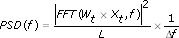# Using the Periodogram Method (Advanced Signal Processing Toolkit)

LabVIEW 2014 Advanced Signal Processing Toolkit Help

Edition Date: June 2014

Part Number: 372656C-01

»View Product InfoDownload Help (Windows Only)

The periodogram method is the most common nonparametric method for computing the power spectral density (PSD) of a time series. This method computes the PSD with the fast Fourier transform (FFT) according to the following equation:where Xt is a time series L is the number of samples in the time series Wt is the applied window function Δf is the frequency interval

The applied window function reduces spectral leakage in the estimation, but the window function also decreases the frequency resolution at the same time.

Use the TSA Periodogram VI to compute the PSD of a time series. If a time series contains non-periodic signal components or periodic components that are not sampled synchronously, the value of the resulting PSD computed by this VI may have a large variance at each frequency.